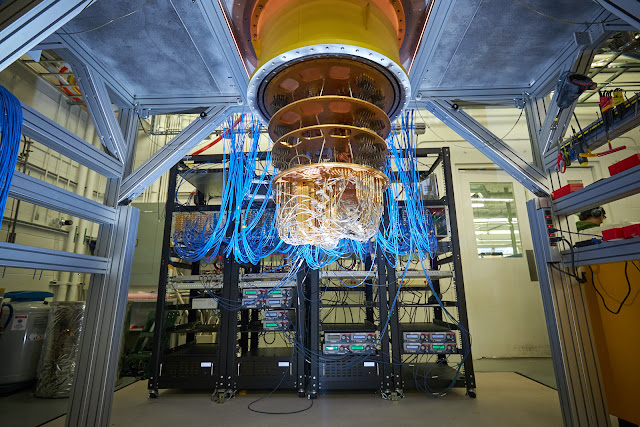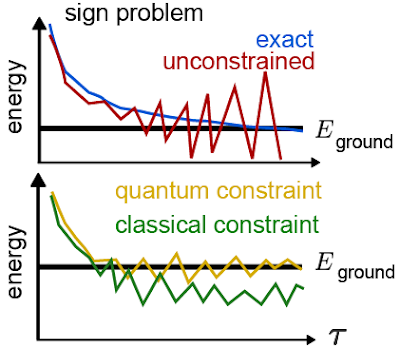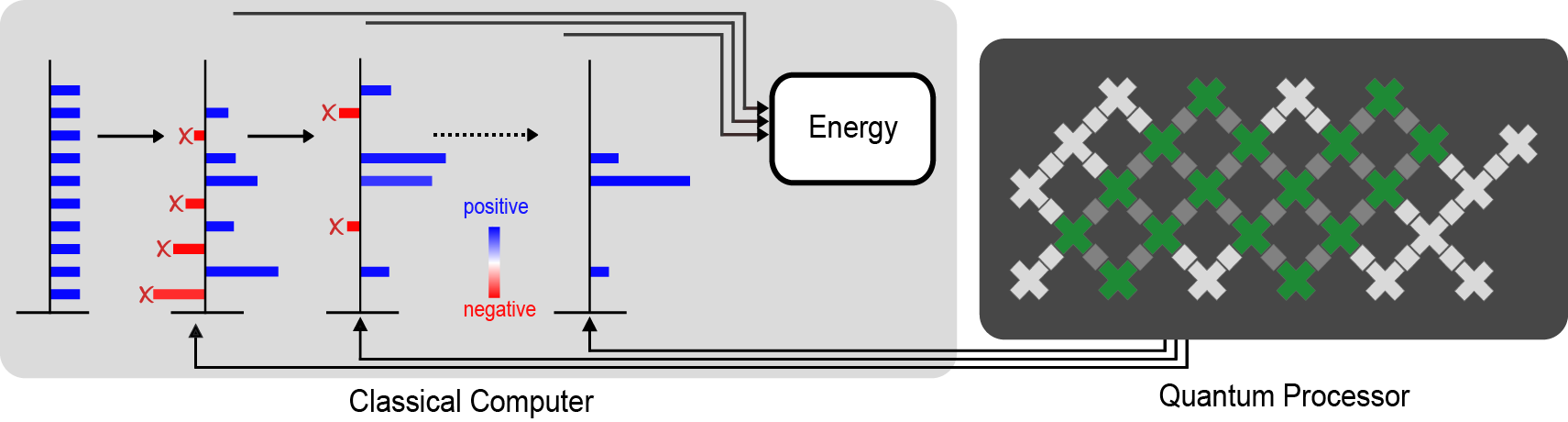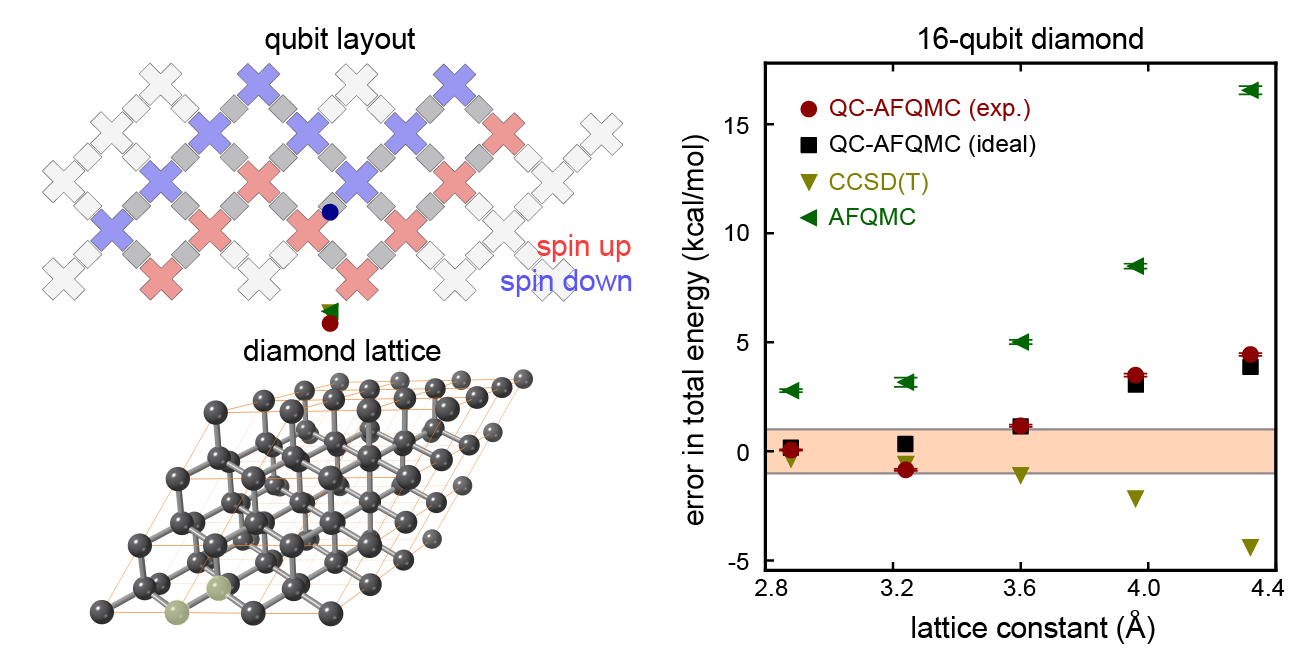# Hybrid Quantum Algorithms for Quantum Monte Carlo

The intersection between the computational difficulty and practical importance of quantum chemistry challenges run on quantum computers has long been a focus for Google Quantum AI. We’ve experimentally simulated simple models of chemical bonding, high-temperature superconductivity, nanowires, and even exotic phases of matter such as time crystals on our Sycamore quantum processors. We’ve also developed algorithms suitable for the error-corrected quantum computers we aim to build, including the world’s most efficient algorithm for large-scale quantum computations of chemistry (in the usual way of formulating the problem) and a pioneering approach that allows for us to solve the same problem at an extremely high spatial resolution by encoding the position of the electrons differently.

Despite these successes, it is still more effective to use classical algorithms for studying quantum chemistry than the noisy quantum processors we have available today. However, when the laws of quantum mechanics are translated into programs that a classical computer can run, we often find that the amount of time or memory required scales very poorly with the size of the physical system to simulate.

Today, in collaboration with Dr. Joonho Lee and Professor David Reichmann at Colombia, we present the Nature publication “Unbiasing Fermionic Quantum Monte Carlo with a Quantum Computer”, where we propose and experimentally validate a new way of combining classical and quantum computation to study chemistry, which can replace a computationally-expensive subroutine in a powerful classical algorithm with a “cheaper”, noisy, calculation on a small quantum computer. To evaluate the performance of this hybrid quantum-classical approach, we applied this idea to perform the largest quantum computation of chemistry to date, using 16 qubits to study the forces experienced by two carbon atoms in a diamond crystal. Not only was this experiment four qubits larger than our earlier chemistry calculations on Sycamore, we were also able to use a more comprehensive description of the physics that fully incorporated the interactions between electrons.Google’s Sycamore quantum processor. Photo Credit: Rocco Ceselin.

## A New Way of Combining Quantum and Classical

Our starting point was to use a family of Monte Carlo techniques (projector Monte Carlo, more on that below) to give us a useful description of the lowest energy state of a quantum mechanical system (like the two carbon atoms in a crystal mentioned above). However, even just storing a good description of a quantum state (the “wavefunction”) on a classical computer can be prohibitively expensive, let alone calculating one.

Projector Monte Carlo methods provide a way around this difficulty. Instead of writing down a full description of the state, we design a set of rules for generating a large number of oversimplified descriptions of the state (for example, lists of where each electron might be in space) whose average is a good approximation to the real ground state. The “projector” in projector Monte Carlo refers to how we design these rules — by continuously trying to filter out the incorrect answers using a mathematical process called projection, similar to how a silhouette is a projection of a three-dimensional object onto a two-dimensional surface.

Unfortunately, when it comes to chemistry or materials science, this idea isn’t enough to find the ground state on its own. Electrons belong to a class of particles known as fermions, which have a surprising quantum mechanical quirk to their behavior. When two identical fermions swap places, the quantum mechanical wavefunction (the mathematical description that tells us everything there is to know about them) picks up a minus sign. This minus sign gives rise to the famous Pauli exclusion principle (the fact that two fermions cannot occupy the same state). It can also cause projector Monte Carlo calculations to become inefficient or even break down completely. The usual resolution to this fermion sign problem involves tweaking the Monte Carlo algorithm to include some information from an approximation to the ground state. By using an approximation (even a crude one) to the lowest energy state as a guide, it is usually possible to avoid breakdowns and even obtain accurate estimates of the properties of the true ground state.Top: An illustration of how the fermion sign problem appears in some cases. Instead of following the blue line curve, our estimates of the energy follow the red curve and become unstable. Bottom: An example of the improvements we might see when we try to fix the sign problem. By using a quantum computer, we hope to improve the initial guess that guides our calculation and obtain a more accurate answer.

For the most challenging problems (such as modeling the breaking of chemical bonds), the computational cost of using an accurate enough initial guess on a classical computer can be too steep to afford, which led our collaborator Dr. Joonho Lee to ask if a quantum computer could help. We had already demonstrated in previous experiments that we can use our quantum computer to approximate the ground state of a quantum system. In these earlier experiments we aimed to measure quantities (such as the energy of the state) that are directly linked to physical properties (like the rate of a chemical reaction). In this new hybrid algorithm, we instead needed to make a very different kind of measurement: quantifying how far the states generated by the Monte Carlo algorithm on our classical computer are from those prepared on the quantum computer. Using some recently developed techniques, we were even able to do all of the measurements on the quantum computer before we ran the Monte Carlo algorithm, separating the quantum computer’s job from the classical computer’s.A diagram of our calculation. The quantum processor (right) measures information that guides the classical calculation (left). The crosses indicate the qubits, with the ones used for the largest experiment shaded green. The direction of the arrows indicate that the quantum processor doesn’t need any feedback from the classical calculation. The red bars represent the parts of the classical calculation that are filtered out by the data from the quantum computer in order to avoid the fermion sign problem and get a good estimate of properties like the energy of the ground state.

This division of labor between the classical and the quantum computer helped us make good use of both resources. Using our Sycamore quantum processor, we prepared a kind of approximation to the ground state that would be difficult to scale up classically. With a few hours of time on the quantum device, we extracted all of the data we needed to run the Monte Carlo algorithm on the classical computer. Even though the data was noisy (like all present-day quantum computations), it had enough signal that it was able to guide the classical computer towards a very accurate reconstruction of the true ground state (shown in the figure below). In fact, we showed that even when we used a low-resolution approximation to the ground state on the quantum computer (just a few qubits encoding the position of the electrons), the classical computer could efficiently solve a much higher resolution version (with more realism about where the electrons can be).Top left: a diagram showing the sixteen qubits we used for our largest experiment. Bottom left: an illustration of the carbon atoms in a diamond crystal. Our calculation focused on two atoms (the two that are highlighted in translucent yellow). Right: A plot showing how the error in the total energy (closer to zero is better) changes as we adjust the lattice constant (the spacing between the two carbon atoms). Many properties we might care about, such as the structure of the crystal, can be determined by understanding how the energy varies as we move the atoms around. The calculations we performed using the quantum computer (red points) are comparable in accuracy to two state-of-the-art classical methods (yellow and green triangles) and are extremely close to the numbers we would have gotten if we had a perfect quantum computer rather than a noisy one (black points). The fact that these red and black points are so close tells us that the error in our calculation comes from using an approximate ground state on the quantum computer that was too simple, not from being overwhelmed by noise on the device.

Using our new hybrid quantum algorithm, we performed the largest ever quantum computation of chemistry or materials science. We used sixteen qubits to calculate the energy of two carbon atoms in a diamond crystal. This experiment was four qubits larger than our first chemistry calculations on Sycamore, we obtained more accurate results, and we were able to use a better model of the underlying physics. By guiding a powerful classical Monte Carlo calculation using data from our quantum computer, we performed these calculations in a way that was naturally robust to noise.

We’re optimistic about the promise of this new research direction and excited to tackle the challenge of scaling these kinds of calculations up towards the boundary of what we can do with classical computing, and even to the hard-to-study corners of the universe. We know the road ahead of us is long, but we’re excited to have another tool in our growing toolbox.

## Acknowledgements

I’d like to thank my co-authors on the manuscript, Bryan O’Gorman, Nicholas Rubin, David Reichman, Ryan Babbush, and especially Joonho Lee for their many contributions, as well as Charles Neill and Pedram Rousham for their help executing the experiment. I’d also like to thank the larger Google Quantum AI team, who designed, built, programmed, and calibrated the Sycamore processor.